Absolute Value
Exponents
Order of Operations
Distributive Property
Fractions
Algebraic Expressions
Write the expression
100

Find the absolute value of:

|-10|100

When you see the following expression, what is the big number called and what is the little number on top of it called?

24

100

Simplify the following expression: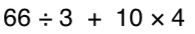100

8(4+2) = ?

What is 48?

100

Solve:

1/2 + 1/4

3/4

100

How many terms are in the expression

4y + 5x - 2 + 2y - -3x

5

100

The sum of 9 and a number

9 + x

200

What is greater:

|-6| or |3|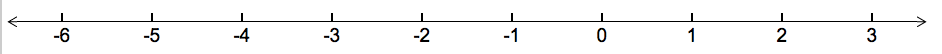The absolute value of a number is the number's distance from zero.

What is l-6 l?

200

How can you rewrite 9in expanded form?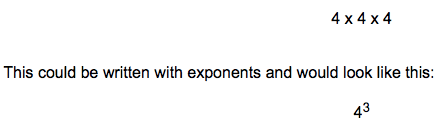What is 9x9?

200

Simplify the following expression: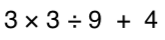200

5(x-3)

What is 5x-15?

200

Convert to an improper fraction

6 2/3 =

20/3

200

What are the Coefficients in the following expression?

4y + 5x - 2 + 2y - 3x

4, 5, 2, 3

200
Half of a number

x -:2

300

Place the following in order from smallest number, to biggest number:

|-20|, 18, |12|, |-14|, 8

What is 8, l 12 l, l -14 l, 18, l-20 l?

The absolute value of a number is the number's distance from zero.

300

How can you rewrite the following in exponential form?

7 x 7 x 7 x 7 x 7What is 75?

300

3 + 42 * (3 + 2)

what is 213?

300

7+9(2x-3)

What is 18x-20?

300

4/5 * 3/7 =

12/35

300

What is the degree of the function

3x^2-4x-2-4x^3

3

300

The difference between three times a number and 5

3x-5

400

What is:

|-7| + |-2|

The absolute value of a number is the number's distance from zero.

What is l -9 l ?

400

What is the value of:

24

400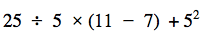What is 45?

400

3(x-10)

What is 3x - 30?

400

Solve:

2 5/7 - 1 3/7

1 2/7

400

What are the Variables in the following expression?

4 + 8x - 3 + 2y - 3x

y, x

400

A number squared increased by five cubed

x^2+5^3

500

2 x |-5| + |4| - 14

Remember to use your order of operations.

What is 0?

500

What is the value of:

23 + 32 - 42

500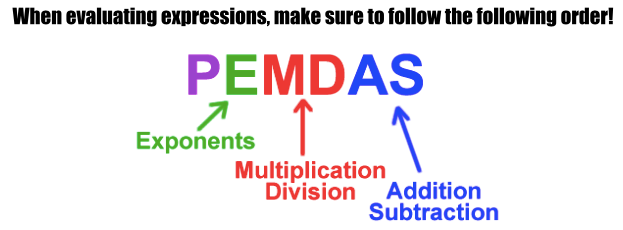What is 30?

500

6(x-3x) +4

What is 6x - 18x + 4 = -12x + 4

500

8/9 -: 3/5 =

8/9 * 5/3 = 40/27 or 1 13/27

500

Combine like terms in the following expression

5v+ 4y + 5x - 2 + 2y - 3x - 2v2

3v2 + 6y + 2x - 2

500

A number multiplied by seven is split into three equal groups.

7x-:3

Click to zoom